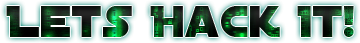# Image Data Function Record

This header describes the physical meaning of the Image included in the LRIT File. It may contain calibration data depending on the image type.

Name Type Description
Data string Data

### Table 8 - Image Data Function Record Fields

The Data field is consisted by several map values in `key := value` format with some special meanings.

Key Description
\$HALFTONE Specifies a Halftone Grayscale Data.
\$DISCRETE Specifies a Discrete Type that each value has a specific meaning
\$OVERLAY Specifies a Overlay Data
_NAME Name of the image
_UNIT Unit used for the data
a number A map to defined values

This is usually used for marking overlays or calibration data. One great example to explain is a thermal infrared channel that has a map for each pixel value to it’s corresponding temperature. In this case, there will be a \$HALFTONE field defined with the number of bits that are relative to the map, a _UNIT field with the type of unit, for example Degrees Kelvin, and then several `number := number` maps representing each value. For example:

``````\$HALFTONE:=8
_NAME:=Calibrated Infrared
_UNIT:=Degree Kelvin
0:=330
1:=329.5
2:=329
3:=328.5
4:=328
5:=327.5
6:=327
7:=326.5
8:=326
9:=325.5
10:=325
11:=324.5
12:=324
13:=323.5
(...)
``````

A pixel with value 11 mean its temperature is 324.5 Degrees Kelvin.